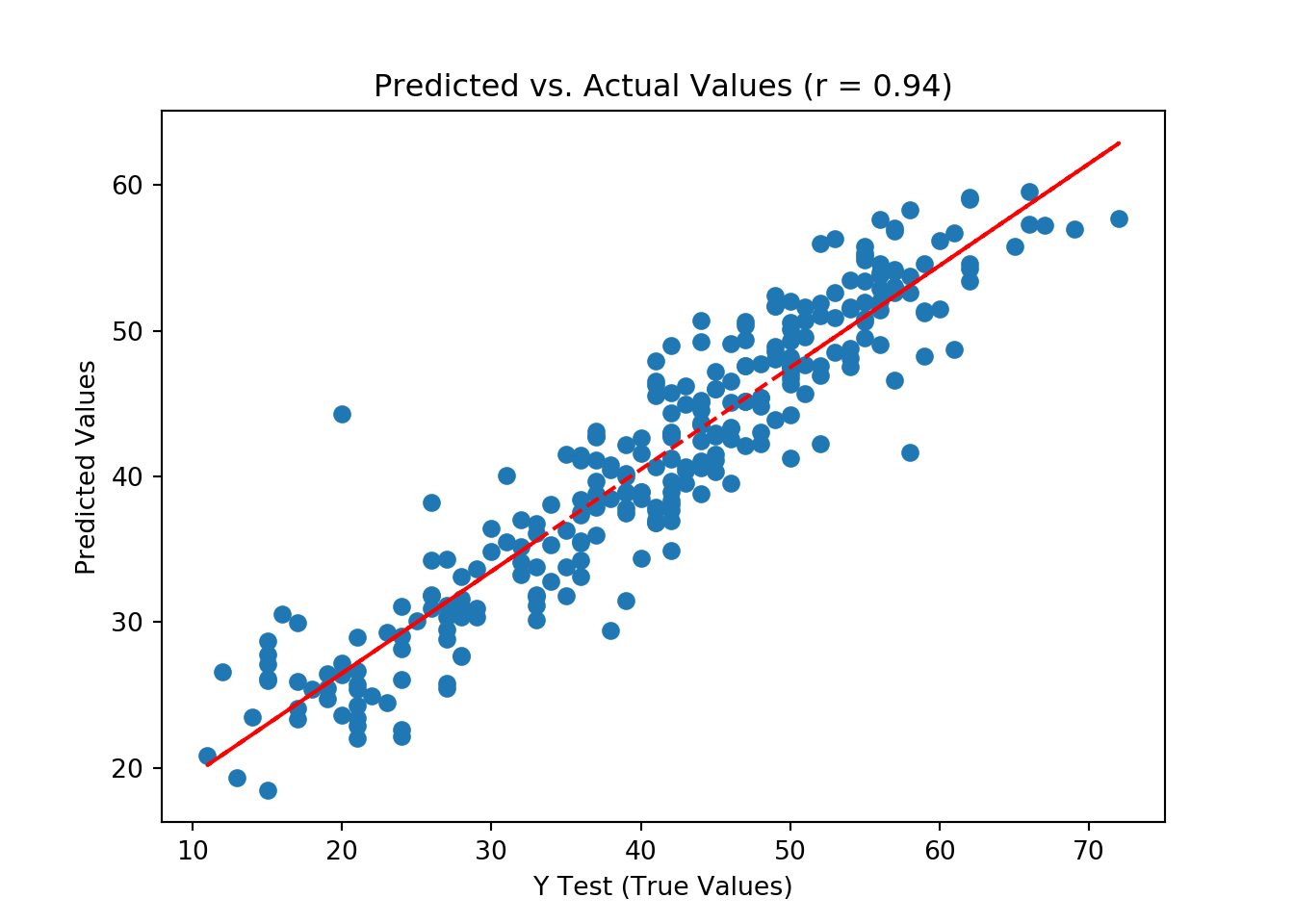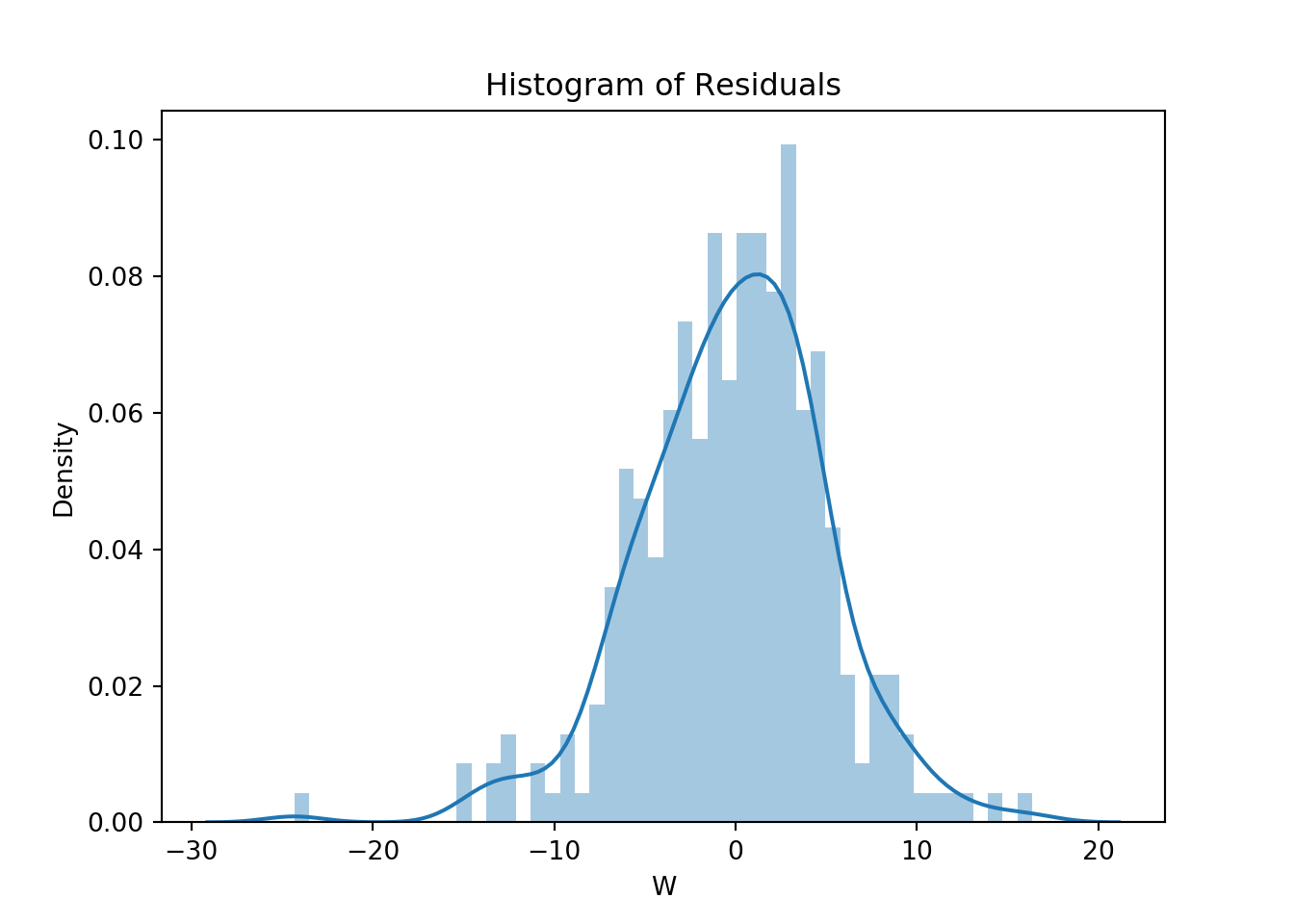# Tune Random Forest Regressor in Pipeline

### Import dependencies

import pandas as pd
from sklearn.model_selection import train_test_split
from sklearn.ensemble import RandomForestRegressor
from sklearn.preprocessing import StandardScaler
from sklearn.pipeline import Pipeline
from sklearn.model_selection import RandomizedSearchCV
import time
import matplotlib.pyplot as plt
import seaborn as sns
from sklearn import metrics
import numpy as np
from scipy.stats import pearsonr, shapiro

### Import data

df = pd.read_csv('dt_NBA_reg.csv')

### Save the DV as DV

DV = 'W'

### Dummy code categorical variables

final_data = pd.get_dummies(df, drop_first=True)

### Save IVs (X) and DV (y) and then split them into testing/training

X = final_data.drop(DV, axis = 1)
y = final_data[DV]
# Create train and test sets
X_train, X_test, y_train, y_test = train_test_split(X, y, test_size=0.33, random_state=42)

### Set up the steps for a pipeline

steps = [('scaler', StandardScaler()), ('Forest', RandomForestRegressor())]

### Setup the pipeline

pipeline = Pipeline(steps)

### Specify the hyperparameter space

parameters = {'Forest__n_estimators': [200, 400, 600, 800, 1000, 1200, 1400, 1600, 1800, 2000],
'Forest__max_features': ['auto', 'sqrt','log2',None],
'Forest__min_samples_split': [2, 5, 10],
'Forest__min_samples_leaf': [1, 2, 4],
'Forest__bootstrap': [True, False],
'Forest__warm_start': [True, False]}

### Instantiate the GridSearchCV model

model = RandomizedSearchCV(pipeline, parameters, n_iter=10, scoring='explained_variance', cv=4)

### Time the tuning (start)

start_time = time.time()

### Fit to the training set

model.fit(X_train, y_train)

### Time the tuning (end)

elapsed_time = (time.time() - start_time)/60
print('Time to tune the model: {} min.'.format(elapsed_time))
## Time to tune the model: 1.9312451163927713 min.

### Print the tuned parameters

print('Tuned Model Parameters: {}'.format(model.best_params_))
## Tuned Model Parameters: {'Forest__warm_start': True, 'Forest__n_estimators': 1400, 'Forest__min_samples_split': 5, 'Forest__min_samples_leaf': 1, 'Forest__max_features': None, 'Forest__bootstrap': True}

### Get predictions

predictions = model.predict(X_test)

### Get scatterplot of actual vs. predicted values

plt.scatter(y_test, predictions)
z = np.polyfit(y_test, predictions, 1)
p = np.poly1d(z)
plt.plot(y_test, p(y_test), 'r--')
plt.xlabel('Y Test (True Values)')
plt.ylabel('Predicted Values')
plt.title('Predicted vs. Actual Values (r = {0:0.2f})'.format(pearsonr(y_test, predictions), 2))
plt.savefig('Rand_For_Reg_Resid')
plt.show()plt.clf()

### Print interpretation of the pearson r

if pearsonr(y_test, predictions) == 1.00:
print('There is a perfect positive linear relationship between the predicted and actual values.')
elif pearsonr(y_test, predictions) >= 0.8:
print('There is a very strong, positive linear relationship between the predicted and actual values.')
elif pearsonr(y_test, predictions) >= 0.6:
print('There is a strong, positive linear relationship between the predicted and actual values.')
elif pearsonr(y_test, predictions) >= 0.4:
print('There is a moderate, positive linear relationship between the predicted and actual values.')
elif pearsonr(y_test, predictions) >= 0.2:
print('There is a weak, positive linear relationship between the predicted and actual values.')
elif pearsonr(y_test, predictions) > 0:
print('There is a very weak, positive linear relationship between the predicted and actual values.')
elif pearsonr(y_test, predictions) == 0:
print('There is no linear relationship between the predicted and actual values.')
elif pearsonr(y_test, predictions) <= -0.8:
print('There is a very strong, negative linear relationship between the predicted and actual values.')
elif pearsonr(y_test, predictions) <= -0.6:
print('There is a strong, negative linear relationship between the predicted and actual values.')
elif pearsonr(y_test, predictions) <= -0.4:
print('There is a moderate, negative linear relationship between the predicted and actual values.')
elif pearsonr(y_test, predictions) <= -0.2:
print('There is a weak, negative linear relationship between the predicted and actual values.')
else: # <= 0 and pearsonr(y_test, predictions) > -0.2
print('There is a very weak, negative linear relationship between the predicted and actual values.')
## There is a very strong, positive linear relationship between the predicted and actual values.

### Print regression metrics

# make metrics into a dataframe
metrics_df = pd.DataFrame({'Metric': ['MAE',
'MSE',
'RMSE',
'R-Squared'],
'Value': [metrics.mean_absolute_error(y_test, predictions),
metrics.mean_squared_error(y_test, predictions),
np.sqrt(metrics.mean_squared_error(y_test, predictions)),
metrics.explained_variance_score(y_test, predictions)]}).round(3)
print(metrics_df)
##       Metric   Value
## 0        MAE   3.969
## 1        MSE  27.024
## 2       RMSE   5.198
## 3  R-Squared   0.840

### Plot histogram of residuals (we want them to be normally distributed)

sns.distplot((y_test - predictions), bins = 50)
## C:\Users\aengland\AppData\Local\CONTIN~1\ANACON~1\lib\site-packages\matplotlib\axes\_axes.py:6499: MatplotlibDeprecationWarning:
## The 'normed' kwarg was deprecated in Matplotlib 2.1 and will be removed in 3.1. Use 'density' instead.
##   alternative="'density'", removal="3.1")
plt.xlabel(DV)
plt.ylabel('Density')
plt.title('Histogram of Residuals')
plt.savefig('Rand_For_Reg_Resid_Hist')
plt.show()plt.clf()

### Check residuals for normality

shapiro_df = pd.DataFrame({'Metric': ['Shapiro W',
'p-value'],
'Value': [shapiro(y_test - predictions),
shapiro(y_test - predictions)]}).round(3)
print(shapiro_df)
##       Metric  Value
## 0  Shapiro W  0.981
## 1    p-value  0.001

### Print the interpretation of the test

if shapiro(y_test - predictions) > 0.05:
print('Fail to reject the null hypothesis. Data is normally distributed.')
else:
print('Null hypothesis is rejected. Data is not normally distributed.')
## Null hypothesis is rejected. Data is not normally distributed.